# Unlevered Beta Formula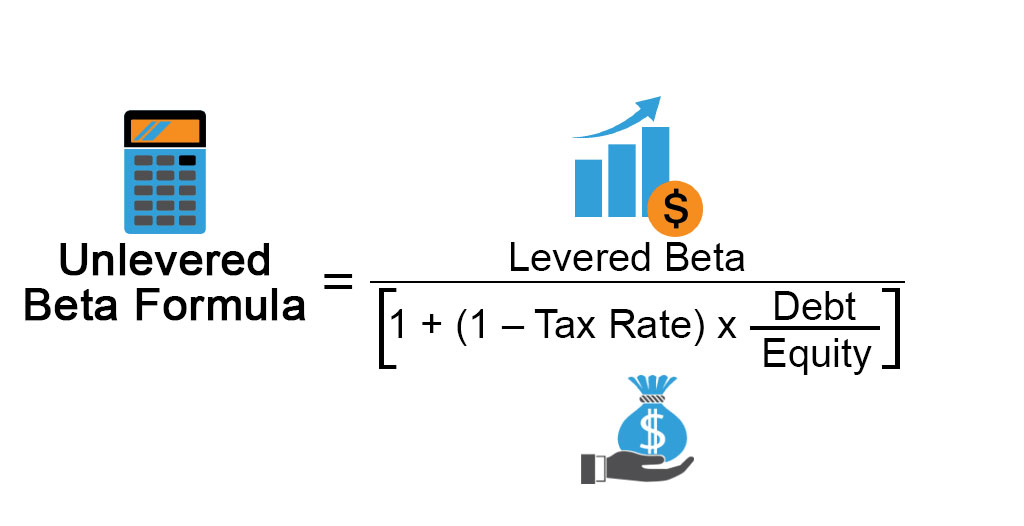• Formula
• Examples
• Calculator

## What is the Unlevered Beta Formula?

The term “unlevered beta” refers to the true systematic risk of a company’s assets from which the financial risk due to debt has been removed and as such, it is also known as asset Beta. In other words, Unlevered Beta is that beta which nullifies the effect of the capital structure of a company on its systematic risk exposure. The formula for unlevered beta can be derived by dividing the levered beta (a.k.a. equity beta) by a factor of 1 plus the product of (1 – tax rate) and the debt-to-equity ratio of the company. Mathematically, it is represented as,

Unlevered Beta = Levered Beta / [1 + (1 – Tax Rate) * (Debt / Equity)]

### Examples of Unlevered Beta Formula (With Excel Template)

Let’s take an example to understand the calculation of Unlevered Beta Formula in a better manner.

You can download this Unlevered Beta Formula Excel Template here – Unlevered Beta Formula Excel Template

#### Unlevered Beta Formula – Example #1

Let us take the example of John Doe who wants to calculate the unlevered beta of a company named GHK Ltd. It is a publicly listed company with a levered beta of 0.8, the total debt of \$200 million and a market capitalization of \$400 million. Help John Calculate the unlevered beta of the company if its effective tax rate is 30%.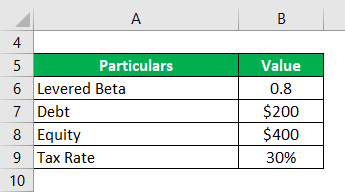Solution:

Unlevered Beta is calculated using the formula given below

Unlevered Beta = Levered Beta / [1 + (1 – Tax Rate) * (Debt / Equity)]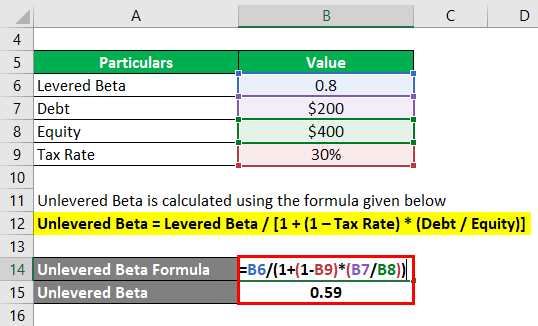•  Unlevered Beta = 0.8 / [(1 + (1 – 30%) * (\$200 million / \$400 million)]
•  Unlevered Beta = 0.59

Therefore, the unlevered beta of GHK Ltd. is 0.59.

#### Unlevered Beta Formula – Example #2

Let us take the example of Apple Inc. to illustrate the concept of unlevered beta for real-life companies. As per the available market information, the 1-year levered beta of the stock of the company is 1.08. As per the latest annual report, non-current term debt and current term debt at \$93.74 billion and \$8.78 billion respectively as on September 29, 2018. On the other hand, the current market capitalization stood at \$922.64 billion. Calculate the unlevered beta of Apple Inc. if the effective tax rate for the year is 24.5%.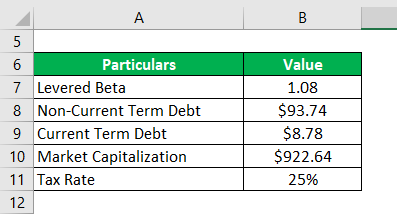Solution:

Debt is calculated using the formula given below

Debt = Non-Current Term Debt + Current Term Debt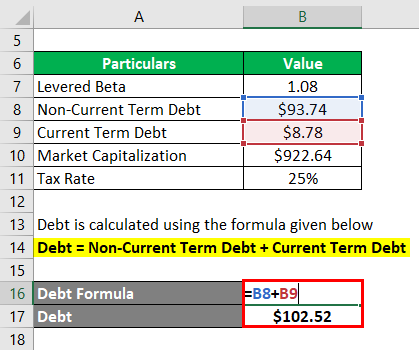• Debt = \$93.74 billion + \$8.78 billion
• Debt= \$102.52 billion

Unlevered Beta is calculated using the formula given below

Unlevered Beta = Levered Beta / [1 + (1 – Tax Rate) * (Debt / Equity)]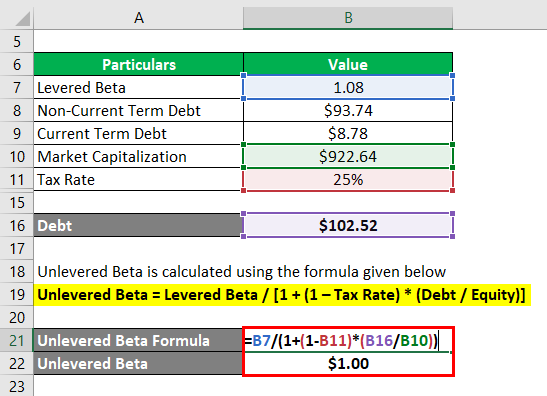• Unlevered Beta = 1.08 / [(1 + (1 – 24.5%) * (\$102.52 billion / \$922.64 billion)]
• Unlevered Beta = 1.00

Based on the given information, the unlevered beta of Apple Inc. stood at 1.00.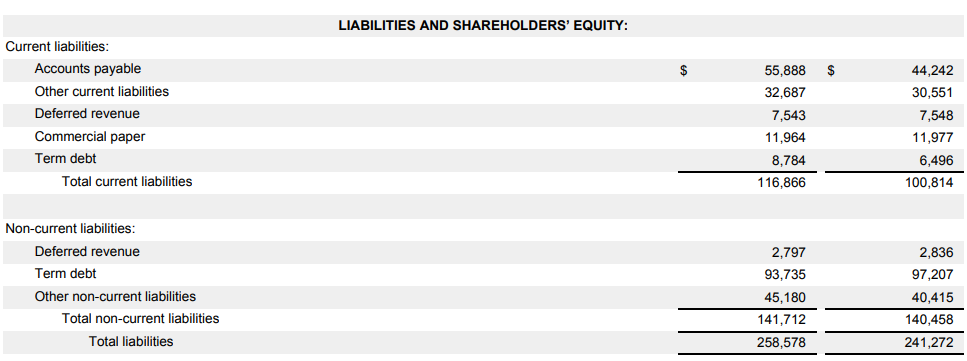#### Unlevered Beta Formula – Example #3

Let us take the example of Samsung Electronics Co. Ltd. and calculate its unlevered beta. As per the available market information, the 1-year levered beta of the stock of the company is 1.47. As per the latest annual report, short-term borrowings, current portion of long-term liabilities and long-term borrowings at \$12.35 billion, \$0.03 billion and \$0.08 billion respectively as on December 31, 2018. Calculate the unlevered beta of the company if its current market capitalization is \$245.92 billion and the corporate tax rate in South Korea is 25%.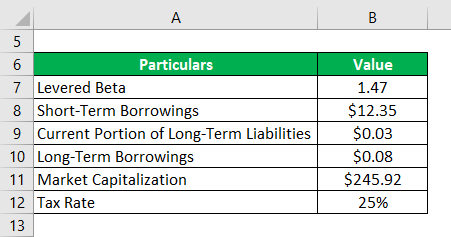Solution:

Debt is calculated using the formula given below

Debt = Short-Term Borrowings + Current Portion of Long-Term Liabilities + Long-Term Borrowings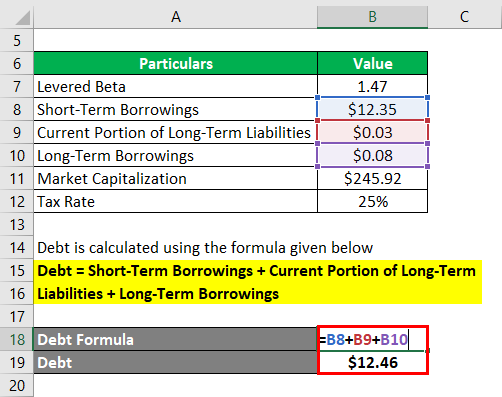• Debt = \$12.35 billion + \$0.03 billion + \$0.08 billion
• Debt = \$12.46 billion

Unlevered Beta is calculated using the formula given below

Unlevered Beta = Levered Beta / [1 + (1 – Tax Rate) * (Debt / Equity)]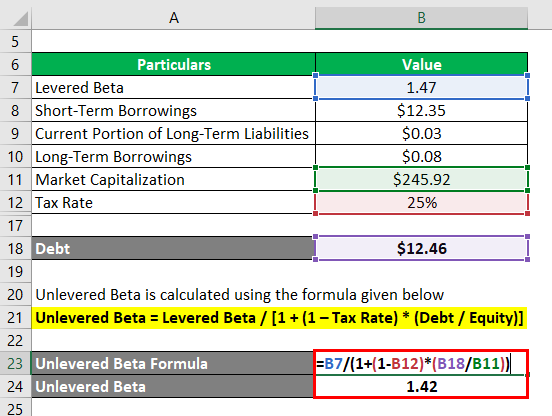• Unlevered Beta = 1.47 / [(1 + (1 – 25%) * (\$12.46 billion / \$245.92 billion)]
• Unlevered Beta = 1.42

Therefore, the unlevered beta of Samsung Electronics Co. Ltd. is 1.42.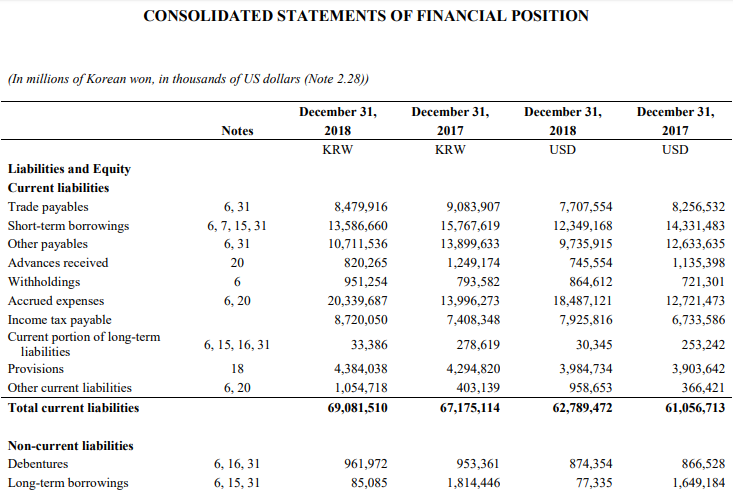### Explanation

The formula for unlevered beta can be computed by using the following steps:

Step 1: Firstly, determine the levered beta of the subject company. Usually, the levered beta of publicly listed companies can be derived from the market.

Step 2: Next, collect the value of the debt of the company which is available from its balance sheet.

Step 3: Next, determine the value of the company’s equity-based on the market capitalization or the trading value of the stock times the number of shares.

Step 4: Next, figure out the effective tax rate applicable to the company either from its income statement or use the corporate tax rate of the country of incorporation. The tax component of the formula can be calculated as 1 minus the tax rate.

Step 5: Next determine the leverage of the company by dividing the debt (step 2) by the equity (step 3) of the company.

Debt-to-Equity Ratio = Debt / Equity

Step 6: Finally, the formula for unlevered beta can be derived by dividing the levered beta (step 1) by a factor of 1 plus the product of (1 – tax rate) (step 4) and the debt-to-equity (step 5) ratio as shown below.

Unlevered Beta = Levered Beta /[1 + (1 – Tax Rate) * (Debt / Equity)]

### Relevance and Use of Unlevered Beta Formula

It is important to understand the concept of unlevered beta because it is predominantly used for a pure-play method which is an approach for determining the cost of equity. In this method, the equity beta of a publicly available comparable company is unlevered using the debt-to-equity ratio of a comparable company. Finally, the levered beta of the subject company is derived by re-leveraging the unlevered beta by using its debt-to-equity ratio.

### Unlevered Beta Formula Calculator

You can use the following Unlevered Beta Formula Calculator

 Levered Beta Tax Rate Debt Equity Unlevered Beta

 Unlevered Beta = Levered Beta / [1 + (1 -Tax Rate) * (Debt / Equity)] 0 / [1 + (1 -0) * (0 / 0)] = 0

### Recommended Articles

This is a guide to Unlevered Beta Formula. Here we discuss how to calculate Unlevered Beta Formula along with practical examples. We also provide an Unlevered Beta calculator with a downloadable excel template. You may also look at the following articles to learn more –

1. Formula for Predetermined Overhead Rate
2. How to Calculate Nominal Interest Rate?
3. Examples of Manufacturing Overhead Formula
4. Calculation for Vector Cross Product Formula

The post Unlevered Beta Formula appeared first on EDUCBA.

This post first appeared on Best Online Training & Video Courses | EduCBA, please read the originial post: here